# DIMENSION REDUCTION OF FPK EQUATION FOR VELOCITY RESPONSE ANALYSIS OF STRUCTURES SUBJECTED TO ADDITIVE NONSTATIONARY EXCITATIONS1)

Rui Zhenmei, Chen Jianbing2)State Key Laboratory of Disaster Reduction in Civil Engineering & College of Civil Engineering, Tongji University, Shanghai 200092, China

Abstract

The nonlinear response analysis of structures subjected to random excitation is a highly challenging problem. The solution of FPK equation provides exact solutions to these problems. However, for nonlinear multi-degree-of-freedom systems, the direct solutions of the FPK equation is prohibitively difficult. Actually, the numerical solutions are strictly limited by the dimension of the equation, while the analytical solutions are available only for very few specific systems, and most of them are steady-state solution. Therefore, reducing the dimension of the FPK equation is an important way to solve the high-dimensional nonlinear dynamic response analysis problem. In the present paper, for the nonstationary response analysis of multi-degree-of-freedom nonlinear structures subjected to amplitude-modulated additive white noise, the high-dimensional FPK equation in terms of the joint probability density function is reduced in dimension. For the probability density function of velocity response, it is converted to a one-dimensional FPK-like equation through introducing the equivalent drift coefficient. The method of conditional mean function estimate is suggested to construct the equivalent drift coefficient. Afterwards, the numerical results of probability density function of velocity can be obtained by applying the path integration method to solve the dimension-reduced FPK-like equation. The accuracy and efficiency of the proposed method are discussed and verified through the numerical examples, including the non-stationary response analysis of velocity of a single-degree-of-freedom Rayleigh oscillator, a ten-story linear shear frame structure and a nonlinear shear frame structure subjected to amplitude-modulated additive white noise.

Keywords： amplitude modulation ; white noise ; FPK equation ; dimension-reduction ; nonlinear

0

Rui Zhenmei, Chen Jianbing. DIMENSION REDUCTION OF FPK EQUATION FOR VELOCITY RESPONSE ANALYSIS OF STRUCTURES SUBJECTED TO ADDITIVE NONSTATIONARY EXCITATIONS1)[J]. Chinese Journal of Theoretical and Applied Mechanics, 2019, 51(3): 922-931 https://doi.org/10.6052/0459-1879-18-411

## 1 高维FPK方程降维

### 1.1 幅值调制的白噪声激励系统及其非平稳响应的FPK方程

$$\tilde {\xi }(t) = g(t)\xi (t) (1)$$

$$E[\xi (t)] = 0,\;\;E[\xi (t)\xi ({t}')] = D\delta (t - {t}') (2)$$

$${\pmb M}\ddot{\pmb X} + {\pmb f} ({\pmb X}, \dot{\pmb X} ) = -{\pmb M} {\pmb 1}\tilde {\xi }(t) (3)$$

$$\dot{\pmb Y} = {\pmb A}\left( {{\pmb Y},t} \right) +{\pmb B}g(t)\xi (t) (4)$$

$${\pmb A}\left( {{\pmb Y},t} \right) = \left\{ \!\!\begin{array}{l} {\pmb V} -{\pmb M}{\pmb f}^{ - 1}\left( {{\pmb X},{\pmb V}} \right) \end{array} \right\},, \ {\pmb B} = - \left\{ \!\!\begin{array}{l} {\bf 0}_{n\times 1} {\pmb I} \end{array} \right\} (5)$$

$${\pmb Y}(t) = {\pmb A}({\pmb Y},t) t + {\pmb B}g(t) W(t) (6)$$

$$\dfrac{\partial p_{ Y} ({\pmb y},t)}{\partial t} = - \sum_{j = 1}^{2n} \dfrac{\partial [{\pmb A}_j ({\pmb y},t)p({\pmb y},t)]}{\partial y_j }+ \dfrac{g^2(t)}{2}\sum_{i = 1}^{2n} \sum_{j = 1}^{2n} \sigma _{ij} \dfrac{\partial ^2p({\pmb y},t)}{\partial y_i \partial y_j } (7)$$

$${\pmb \sigma } = {\pmb B}{\pmb B}^{\rm T}D (8)$$

FPK方程(7)的边界条件一般为

$$\left. {p_{ Y} ({\pmb y},t)} \right|_{y_j \to \infty } = 0 (9)$$

$$p_{ Y} ({\pmb y},t) = \delta ({\pmb y} - {\pmb y}_0 ) (10)$$

### 1.2 FPK方程的降维

$$\dfrac{\partial p_{Y_l } (y_l ,t)}{\partial t} = - \dfrac{\partial J_l (y_l,t)}{\partial y_l } + \dfrac{\sigma _{ll} g^2(t)}{2}\dfrac{\partial^2 p_{Y_l } (y_l ,t)} {\partial y_l^2 } (11)$$

$p_{Y_l } (y_l ,t) = \int_{ - \infty }^\infty { \cdots \int_{ - \infty }^\infty {p_{ Y} ({\pmb y},t)} } y_1 \cdots y_{l - 1} y_{l + 1} \cdots y_{2n}$

$J_l (y_l ,t)$为$y_l$方向的概率通量

$$J_l (y_l ,t) = \int_{ - \infty }^\infty { \cdots \int_{ - \infty }^\infty {A_l ({\pmb y},t)} } \cdot p_{ Y} ( {\pmb y},t ) \qquad y_1 \cdots y_{l - 1} y_{l + 1} \cdots y_{2n} (12)$$

$$a^{\rm eq}(y_l ,t) = \dfrac{J_l (y_l ,t)}{p_{Y_l } (y_l ,t)} (13)$$

$$\dfrac{\partial p_{Y_l } (y_l ,t)}{\partial t} = - \dfrac{\partial a^{eq}(y_l ,t)p^2_{Y_l } (y_l ,t)}{\partial y_l } + \qquad \dfrac{\sigma _{ll} g^2(t)}{2}\dfrac{\partial^2 p_{Y_l } (y_l ,t)}{\partial y_l^2 } (14)$$

### 1.3 等价漂移系数的意义

$a^{\rm eq}(y_l ,t) =$

$\quad \dfrac{\int_{ - \infty }^\infty { \cdots \int_{ - \infty }^\infty {A_l ({\pmb y},t)} } p_{ Y} ( {\pmb y},t ) y_1 \cdots y_{l - 1} y_{l + 1} \cdots y_{2n} }{p_{Y_l } (y_l ,t)} =$

$\quad \int_{ - \infty }^\infty { \!\cdots\! \int_{ - \infty }^\infty \!{A_l ( {\pmb y},t)} } \dfrac{p_{ Y} ( {\pmb y},t ) }{p_{Y_l } (y_l ,t)} y_1 \cdots y_{l - 1} y_{l + 1} \cdots y_{2n} =$

$\quad \int_{ - \infty }^\infty { \cdots \int_{ - \infty }^\infty A_l ( {\pmb y},t) } p_{{ Y}\vert Y_l } ( {\pmb y},t\vert Y_l = y_l )$

$\quad y_1 \cdots y_{l - 1} y_{l + 1} \cdots y_{2n} = E[A_l ( {\pmb Y},t\vert Y_l = y_l ) ] (15)$

## 2 降维FPK型方程求解的数值方法

### 2.1 等价漂移系数的确定

(1) 通过数值模拟生成若干(例如$m$个)白噪声样本$\{\xi ^{(k)}(t)\}_{k = 1}^m$,将其进行幅值调制、即代入式(4)中,进行确定性系统分析,获得一系列响应$\{{\pmb Y}^{(k)}(t)\}_{k = 1}^m$及其分量$\{Y_l^{(k)} (t)\}_{k = 1}^m$,同时可以获得相应的漂移系数样本值$\{A_l^{(k)} (t) = A_l ({\pmb Y}^{(k)}(t),t)\}_{k = 1}^m$.

(2) 对于时刻$t$,可以获得$m$个数据对$\{(y_l^{(k)} ,A_l^{(k)} )\}_{k = 1}^m$,其中$y_l^{(k)} = Y_l^{(k)} (t)$.

(3) 根据二维数据对$\{(y_l^{(k)} , A_l^{(k)} )\}_{k = 1}^m$,估计条件均值函数,由此给出等价漂移系数$a^{\rm eq}(y_l ,t)$. 一般情况下,可以采用数理统计中的诸多方法进行条件均值估计. 例如,当数据较多、而对条件均值函数的数学形式信息较少时,可采用局域平均法;当对条件均值函数的数学形式有清楚的认识时,则可采用最小二乘法获得条件均值函数估计的满意结果.

### 2.2 降维FPK型方程的路径积分求解

$$p_{Y_l } (y,t_{j + 1} ) = \int_{ - \infty }^\infty {p_{Y_l } (y,t_{j + 1} \vert {y}',t_j ) \cdot p_{Y_l } ({y}',t_j ) {y}'} (16)$$

$$p_{Y_l } (y,t_{j + 1} \vert {y}',t_j ) = \dfrac{1}{\sqrt {2\pi \sigma _{ll} \tau } g(t_j )} \cdot \qquad \exp \left\{ { - \dfrac{(y - {y}' - \tau a^{\rm eq}({y}',t_j ))^2}{2\sigma _{ll} \tau g^2(t_j )}} \right\} (17)$$

$$p_{Y_l } (y,0) = \delta (y - y_{l0} ) (18)$$

## 3 典型实例分析

$$g(t) = C \cdot \left( { {\rm e}^{ - B_1 t} - {\rm e}^{ - B_2 t} + \dfrac{1}{3}} \right) (19)$$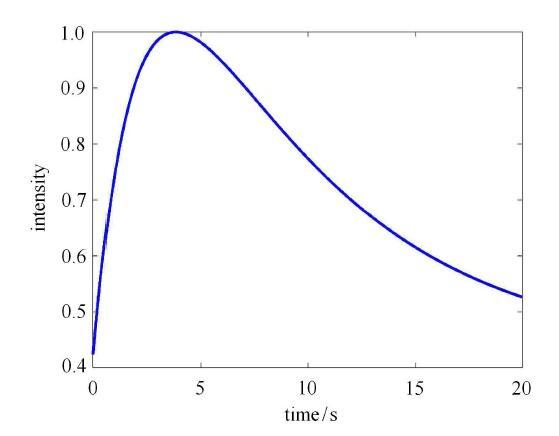Fig. 1   Modulation function versus time

### 3.1 单自由度Rayleigh振子的非平稳速度响应概率密度函数

Rayleigh振子系统具有非线性阻尼,在具有幅值调制的白噪声激励下其运动方程为

$$\ddot {X} + 2\zeta _0 \omega _0 ( - 1 + \varepsilon \dot {X}^2)\dot {X} + \omega _0^2 X = g(t)\xi (t) (20)$$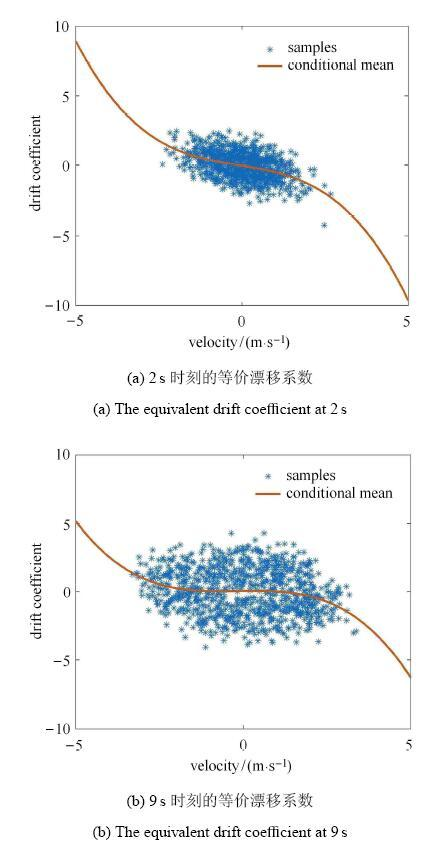Fig. 2   The equivalent drift coefficient at typical time instants (conditional mean function)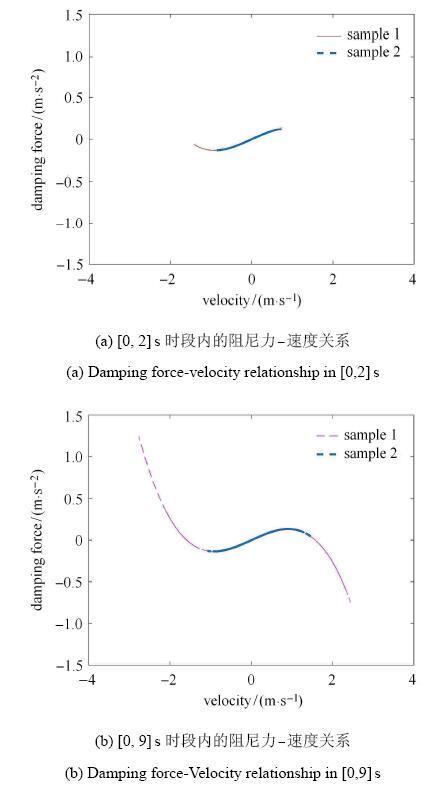Fig. 3   Damping force-Velocity curve in typical time periods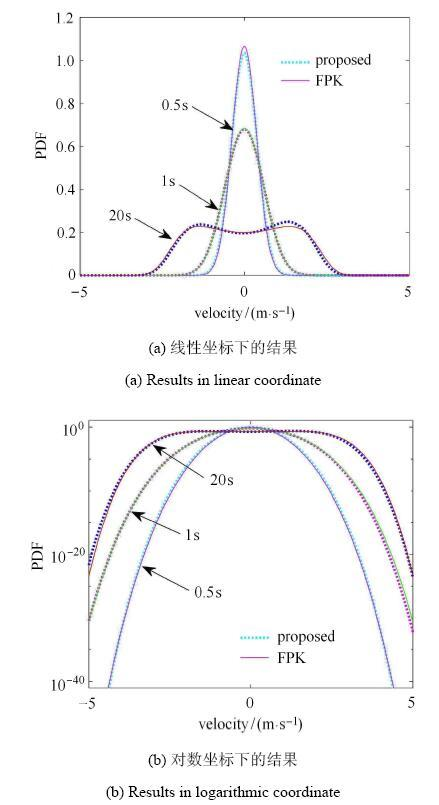Fig. 4   Probability density function solution of velocity response at typical time instants

### 3.2 十层线性剪切型框架结构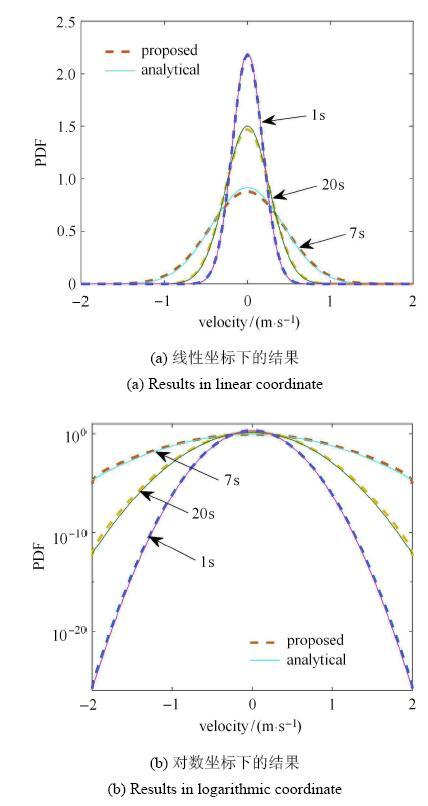Fig. 5   Probability density function solutions at typical instants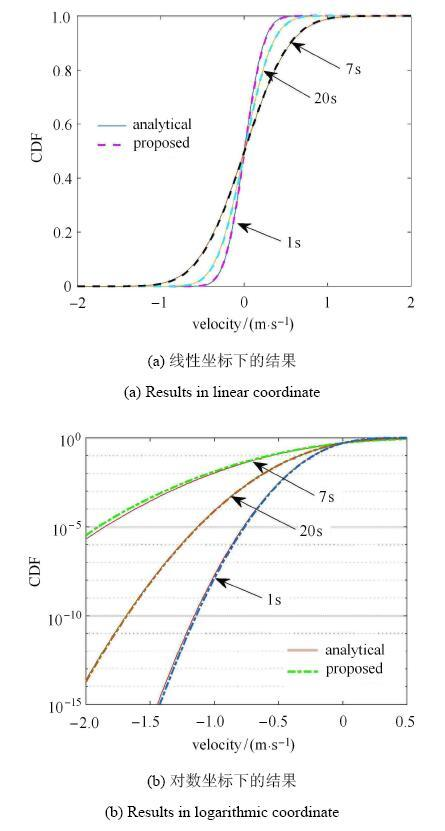Fig. 6   Cumulative distribution function solutions at typical instants

### 3.3 具有非线性恢复力的十层剪切型框架结构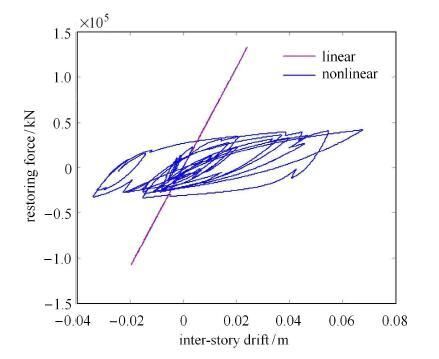Fig. 7   Typical sample of Inter-story drift-Restoring force curve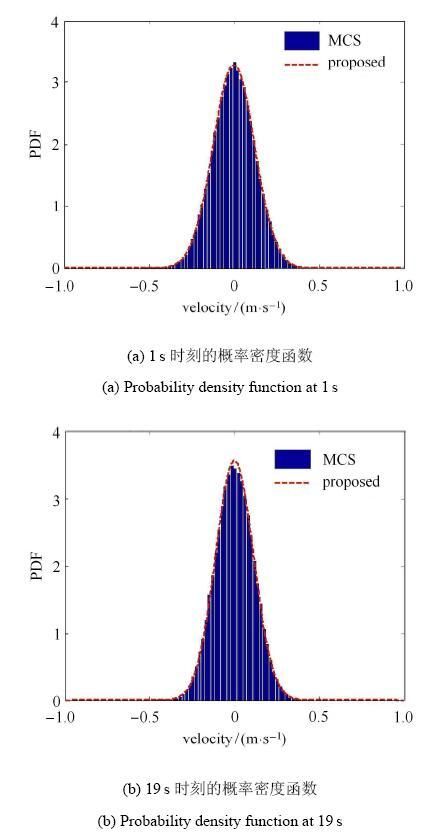Fig. 8   Probability density function solutions of velocity response of the nonlinear structure at typical instants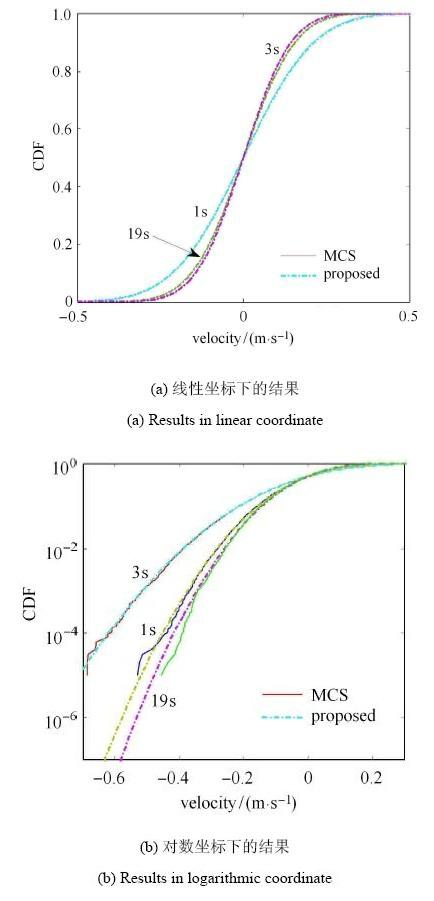Fig. 9   Cumulative distribution function solutions of velocity response of the nonlinear structure at typical time instants

## 4 结 论

(1)等价漂移系数就是原高维系统漂移系数的条件均值函数. 等价漂移系数的形式与物理系统中的非线性形式有关. 为了构造数值稳健的等价漂移系数,一般需要10$^{2}$~10$^{3}$量级的确定性分析.

(2)对幅值调制的加性白噪声激励下线性系统的非平稳速度响应,采用本文建议方法可以获得几乎精确的概率密度函数,其概率分布函数的尾部精度可达10$^{-15}$甚至更高的量级.

(3)对非线性系统在幅值调制的加性白噪声激励下的速度响应,采用本文建议方法亦可获得很高的精度. 与Monte Carlo相比,其效率提高2个数量级以上.

The authors have declared that no competing interests exist.

## 参考文献 原文顺序 文献年度倒序 文中引用次数倒序 被引期刊影响因子

  Lin YK.Probabilistic Theory of Structural Dynamics. New York: McGraw-Hill Book Company, 1967 supports all the CNKI file formats;  Cukier RI, Lakatos-Lindenberg K, Shuler KE.Orthogonal polynomial solutions of the Fokker-Planck equation. Journal of Statistical Physics, 1973, 9(2): 137-144 We have tabulated the form of the coefficientsg1(x) andg2(x) as well as the boundary values [a, b] of the Fokker-Planck equation \frac09P(x, t)09t = - \frac0909x[g1 (x)P(x, t)] + \frac09209x2 [g2 (x)P(x, t)],a \leqslant x \leqslant b for which the solution can be written as an eigenfunction expansion in the classical orthogonal polynomials. We also discuss the problem of finding solutions in terms of the discrete classical polynomials for the differential difference equations of stochastic processes.  Lutes LD, Sarkani S.Random Vibrations: Analysis of Structural and Mechanical Systems. Oxford: Elsevier, 2004  Li J, Chen JB.Stochastic Dynamics of Structures. Singapore: John Wiley & Sons, 2009  Roberts JB, Spanos PD.Random Vibration and Statistical Linearization. Chichester: John Wiley & Sons Ltd, 1990  朱位秋. 随机振动. 北京:科学出版社, 1992 (Zhu Weiqiu. Random Vibration.Beijing: Science Press, 1992(in Chinese))  Naess A, Moan T.Stochastic Dynamics of Marine Structures. Cambridge University Press, 2013  Er GK.An improved closure method for analysis of nonlinear stochastic systems. Nonlinear Dynamics, 1998, 17(3): 285-297 The probability density function (PDF) and the mean up-crossing rate (MCR) of the stationary responses of nonlinear stochastic systems excited by white noise is analyzed based on the assumption that the PDF of the responses is approximated with an exponential function of a polynomial in the state variables. Based upon the approximate PDF, a new technique is developed for the approximate PDF solution of Fokker–Planck–Kolmogorov equation, and consequently, the MCR of the system responses is analyzed. Numerical results showed that the approximate PDFs and MCRs approach to the exact ones as the degree of the polynomial increases.  Risken H.The Fokker-Planck Equation: Methods of Solution and Applications. 2nd ed. Berlin: Springer-Verlag, 1989 One of the central problems synergetics is concerned with consists in the study of macroscopic qualitative changes of systems belonging to various disciplines such as physics, chemistry, or electrical engineering. When such transitions from one state to another take place, fluctuations, i.e., random processes, may play an im portant role. Over the past decades it has turned out that the Fokker-Planck equation pro vides a powerful tool with which the effects of fluctuations close to transition points can be adequately treated and that the approaches based on the Fokker Planck equation are superior to other approaches, e.g., based on Langevin equa tions. Quite generally, the Fokker-Planck equation plays an important role in problems which involve noise, e.g., in electrical circuits. For these reasons I am sure that this book will find a broad audience. It pro vides the reader with a sound basis for the study of the Fokker-Planck equation and gives an excellent survey of the methods of its solution. The author of this book, Hannes Risken, has made substantial contributions to the development and application of such methods, e.g., to laser physics, diffusion in periodic potentials, and other problems. Therefore this book is written by an experienced practitioner, who has had in mind explicit applications to important problems in the natural sciences and electrical engineering.  Caughey TK, Ma F.The exact steady-state solution of a class of non-linear stochastic systems. International Journal of Non-Linear Mechanics, 1982, 17(3): 137-142 In this paper exact steady state solutions are constructed for a class of non-linear systems subjected to stochastic excitation. The results are then applied to both classical and non-classical oscillator problems.  Soize C.The Fokker-Planck Equation for Stochastic Dynamical Systems and Its Explicit Steady State Solutions. Singapore: World Scientific, 1994           摘要 This is an analysis of multidimensional nonlinear dissipative Hamiltonian dynamical systems subjected to parametric and external stochastic excitations by the Fokker-Planck equation method.The author answers three types of questions concerning this area. First, what probabilistic tools are necessary for constructing a stochastic model and deriving the FKP equation for nonlinear stochastic dynamical systems? Secondly, what are the main results concerning the existence and uniqueness of an invariant measure and its associated stationary response? Finally, what is the class of multidimensional dySoize, Christian  朱位秋. 非线性随机动力学与控制:Hamilton 理论体系框架. 北京: 科学出版社, 2003 (Zhu Weiqiu.Nonlinear Stochastic Dynamics and Control in Hamiltonian Formulation. Beijing: Science Press, 2003 (in Chinese))  刘俊, 陈林聪, 孙建桥. 随机激励下滞迟系统的稳态响应闭合解. 力学学报, 2017, 49(3): 685-692 滞迟系统属于一类典型的强非线性系统,滞迟力不仅取决于系统的瞬时变形,还与变形历程有关.虽然滞迟系统的随机振动问题已被广泛研究,但至今尚未得到滞迟系统随机响应概率密度函数的精确闭合解.本文运用迭代加权残值法获得了高斯白噪声激励下Bouc-Wen滞迟系统稳态响应概率密度函数的近似闭合解.首先,运用等效线性化法求出系统的稳态高斯概率密度函数;然后以此构造权函数,应用加权残值法求得了系统指数多项式形式的非高斯概率密度函数;最后引入迭代的过程,逐步优化权函数,提高计算所得结果的精度.以随机地震激励下钢纤维陶粒混凝土结构的稳态响应作为算例,其中Bouc-Wen模型的参数是基于拟静力学试验数据,并应用最小二乘法辨识获得.与Monte Carlo模拟结果相比,等效线性化法得到的结果精度较差;由加权残值法得到的结果能够表现出非线性特征,但其精度依然无法令人满意;采用迭代加权残值法得到的近似闭合解与Monte Carlo模拟的结果吻合非常好;对于较强随机激励情形,采用渐进迭代加权残值法具有较高的求解效率,所获得的理论解析解具有较高的精度.结果表明,所获得的近似闭合解不仅对于土木工程领域具有重要的实际应用价值,而且还可作为检验其他非线性系统随机响应预测方法的精度的标准. (Liu Jun, Chen Lincong, Sun Jian-Qiao.The closed-form solution of steady state response of hysteretic system under stochastic excitation. Chinese Journal of Theoretical and Applied Mechanics, 2017, 49(3): 685-692 (in Chinese)) 滞迟系统属于一类典型的强非线性系统,滞迟力不仅取决于系统的瞬时变形,还与变形历程有关.虽然滞迟系统的随机振动问题已被广泛研究,但至今尚未得到滞迟系统随机响应概率密度函数的精确闭合解.本文运用迭代加权残值法获得了高斯白噪声激励下Bouc-Wen滞迟系统稳态响应概率密度函数的近似闭合解.首先,运用等效线性化法求出系统的稳态高斯概率密度函数;然后以此构造权函数,应用加权残值法求得了系统指数多项式形式的非高斯概率密度函数;最后引入迭代的过程,逐步优化权函数,提高计算所得结果的精度.以随机地震激励下钢纤维陶粒混凝土结构的稳态响应作为算例,其中Bouc-Wen模型的参数是基于拟静力学试验数据,并应用最小二乘法辨识获得.与Monte Carlo模拟结果相比,等效线性化法得到的结果精度较差;由加权残值法得到的结果能够表现出非线性特征,但其精度依然无法令人满意;采用迭代加权残值法得到的近似闭合解与Monte Carlo模拟的结果吻合非常好;对于较强随机激励情形,采用渐进迭代加权残值法具有较高的求解效率,所获得的理论解析解具有较高的精度.结果表明,所获得的近似闭合解不仅对于土木工程领域具有重要的实际应用价值,而且还可作为检验其他非线性系统随机响应预测方法的精度的标准.  Park BT, Petrosian V.Fokker-Planck equations of stochastic acceleration: A study of numerical methods. The Astrophysical Journal Supplement Series, 1996, 103: 255           摘要 Stochastic wave-particle acceleration may be responsible for producing suprathermal particles in many astrophysical situations. The process can be described as a diffusion process through the Fokker-Planck equation. If the acceleration region is homogeneous and the scattering mean free path is much smaller than both the energy change mean free path and the size of the acceleration region, then the Fokker-Planck equation reduces to a simple form involving only the time and energy variables. in an earlier paper (Park & Petrosian 1995, hereafter Paper 1), we studied the analytic properties of the Fokker-Planck equation and found analytic solutions for some simple cases. In this paper, we study the numerical methods which must be used to solve more general forms of the equation. Two classes of numerical methods are finite difference methods and Monte Carlo simulations. We examine six finite difference methods, three fully implicit and three semi-implicit, and a stochastic simulation method which uses the exact correspondence between the Fokker-Planck equation and the it5 stochastic differential equation. As discussed in Paper I, Fokker-Planck equations derived under the above approximations are singular, causing problems with boundary conditions and numerical overflow and underflow. We evaluate each method using three sample equations to test its stability, accuracy, efficiency, and robustness for both time-dependent and steady state solutions. We conclude that the most robust finite difference method is the fully implicit Chang-Cooper method, with minor extensions to account for the escape and injection terms. Other methods suffer from stability and accuracy problems when dealing with some Fokker-Planck equations. The stochastic simulation method, although simple to implement, is susceptible to Poisson noise when insufficient test particles are used and is computationally very expensive compared to the finite difference method.  Spencer BF, Bergman LA.On the numerical solution of the Fokker--Planck equation for nonlinear stochastic systems. Nonlinear Dynamics, 1993, 4, 357-72 The finite element method is applied to the solution of the transient Fokker-Planck equation for several often cited nonlinear stochastic systems accurately giving, for the first time, the joint probability density function of the response for a given initial distribution. The method accommodates nonlinearity in both stiffness and damping as well as both additive and multiplicative excitation, although only the former is considered herein. In contrast to the usual approach of directly solving the backward Kolmogorov equation, when appropriate boundary conditions are prescribed, the probability density function associated with the first passage problem can be directly obtained. Standard numerical methods are employed, and results are shown to be highly accurate. Several systems are examined, including linear, Duffing, and Van der Pol oscillators.  Naess A, Hegstad BK.Response statistics of van der Pol oscillators excited by white noise. Nonlinear Dynamics, 1994, 5(3): 287-297 The joint probability density function of the state space vector of a white noise exoited van der Pol oscillator satisfics a Fokker-Planck-Kolmogorov (FPK) equation. The paper describes a numerical procedure for solving the transient FPK equation based on the path integral solution (PIS) technique. It is shown that by combining the PIS with a cubic B-spline interpolation method, numerical solution algorithms can be implemented giving solutions of the FPK equation that can be made accurate down to very low probability levels. The method is illustrated by application to two specific examples of a van der Pol oscillator.  Yu JS, Cai GQ, Lin YK.A new path integration procedure based on Gauss-Legendre scheme. International Journal of Non-Linear Mechanics, 1997, 32(4): 759-768 A new path integration scheme based on the Gauss-Legendre quadrature integration rule is proposed for calculating the probability density of the response of a dynamical system under Gaussian white-noise excitation. The new scheme is capable of producing accurate results of probability density as it evolves with time, including the tail region where the probability level is very low. This low probability region is important for the system reliability estimation.  Naess A, Moe V.Efficient path integration methods for nonlinear dynamic systems. Probabilistic Engineering Mechanics, 2000, 15(2): 221-231 New approaches for numerical implementation of the path integration (PI) method are described. In essence the PI method is a stepwise calculation of the joint probability density function (PDF) of a set of state space variables describing a white noise excited nonlinear dynamic system. The basic idea behind the proposed procedure is to apply a splines interpolation method to the logarithm of the calculated PDF to obtain an accurate representation of the PDF over the whole domain and not only at the chosen grid points. This exploits the fact that the logarithm of the PDF shows a more polynomial behaviour than the PDF itself, and therefore is better adapted to a splines representation. It is demonstrated that the proposed techniques may lead to significantly improved performance in calculating the response statistics of large classes of nonlinear oscillators excited by white or coloured noise when compared to other available implementations of the PI method. An advantage of the new approaches is that they allow time-variant dynamic systems to be analysed without significant increase in computer time. Numerical results for both 2D and 3D problems are presented.  Kougioumtzoglou IA, Spanos PD.An analytical Wiener path integral technique for non-stationary response determination of nonlinear oscillators. Probabilistic Engineering Mechanics, 2012, 28: 125-131           摘要 78 A novel analytical Wiener path integral technique is developed. 78 The non-stationary response PDF of a class of nonlinear oscillators is determined. 78 The technique is based on statistical linearization and on stochastic averaging. 78 A variational formulation is used for determining the most probable path. 78 No need to advance the solution in short time steps.  徐伟. 非线性随机动力学的若干数值方法及应用. 北京: 科学出版社, 2013 (Xu Wei.Numerical Analysis Methods for Stochastic Dynamical System. Beijing: Science Press, 2013 (in Chinese))  Kougioumtzoglou IA, Di Matteo A, Spanos PD, et al.An efficient Wiener path integral technique formulation for stochastic response determination of nonlinear MDOF systems. Journal of Applied Mechanics, 2015, 82(10): 101005 Abstract The recently developed approximate Wiener path integral (WPI) technique for determining the stochastic response of nonlinear/hysteretic multi-degree-of-freedom (MDOF) systems has proven to be reliable and significantly more efficient than a Monte Carlo simulation (MCS) treatment of the problem for low-dimensional systems. Nevertheless, the standard implementation of the WPI technique can be computationally cumbersome for relatively high-dimensional MDOF systems. In this paper, a novel WPI technique formulation/ implementation is developed by combining the “localization” capabilities of the WPI solution framework with an appropriately chosen expansion for approximating the system response PDF. It is shown that, for the case of relatively high-dimensional systems, the herein proposed implementation can drastically decrease the associated computational cost by several orders of magnitude, as compared to both the standard WPI technique and an MCS approach. Several numerical examples are included, whereas comparisons with pertinent MCS data demonstrate the efficiency and reliability of the technique.  Roberts JB, Spanos PD.Stochastic averaging: an approximate method of solving random vibration problems. International Journal of Non-Linear Mechanics, 1986, 21(2): 111-134 Results obtained by applying the method of stochastic averaging to random vibration problems are discussed. This method is applicable to a variety of problems involving the response of lightly damped systems to broad-band random excitations. Solutions pertaining to both linear and non-linear vibrations are reviewed, and it is shown that the technique enables, in the case of parametric excitation, stability criteria to be established. Some results which have been obtained relating to the first-passage reliability problems are also surveyed. Various applications of the theory to engineering problems are outlined.  Huang ZL, Zhu WQ.Stochastic averaging of quasi-integrable Hamiltonian systems under bounded noise excitations. Probabilistic Engineering Mechanics, 2004, 19(3): 219-228 A stochastic averaging method is proposed to predict approximately the response of quasi-integrable Hamiltonian systems to bounded noise excitations. Two cases of external resonance only and both internal and external resonances are considered separately. The reduced averaged Fokker–Planck–Kolmogorov equation is solved by using the combination of the finite difference method and the successive over relaxation method. An example of two coupled Duffing oscillators under bounded noise excitations is given to illustrate the application of the proposed procedure. It is shown that the analytical results agree well with those from digital simulation of original system.  Er GK.Methodology for the solutions of some reduced Fokker-Planck equations in high dimensions. Annals of Physics, 2011, 523(3): 247-258 In this paper, a new methodology is formulated for solving the reduced Fokker-Planck (FP) equations in high dimensions based on the idea that the state space of large-scale nonlinear stochastic dynamic system is split into two subspaces. The FP equation relevant to the nonlinear stochastic dynamic system is then integrated over one of the subspaces. The FP equation for the joint probability density function of the state variables in another subspace is formulated with some techniques. Therefore, the FP equation in high-dimensional state space is reduced to some FP equations in low-dimensional state spaces, which are solvable with exponential polynomial closure method. Numerical results are presented and compared with the results from Monte Carlo simulation and those from equivalent linearization to show the effectiveness of the presented solution procedure. It attempts to provide an analytical tool for the probabilistic solutions of the nonlinear stochastic dynamics systems arising from statistical mechanics and other areas of science and engineering.  陈建兵, 李杰. 结构随机地震反应与可靠度的概率密度演化分析研究进展. 工程力学, 2014 (4): 1-10 结构随机动力反应与可靠度分析对保障工程结构在灾害性动力作用下的安全性至关重要。数十年来,国内外学者进行了大量的研究,取得了丰硕的成果,但在多自由度非线性系统随机动力反应与可靠度分析方面遇到了巨大的困难。概率密度演化理论提供了全新的处理方法。该文在评述已有研究方法的基础上,着重讨论了概率密度演化理论及其在结构随机地震反应与整体可靠性分析应用方面的新进展。 (Chen Jianbing, Li Jie.Probability density evolution method for stochastic seismic response and reliability of structures. Engineering Mechanics, 2014 (4): 1-10 (in Chinese)) 结构随机动力反应与可靠度分析对保障工程结构在灾害性动力作用下的安全性至关重要。数十年来,国内外学者进行了大量的研究,取得了丰硕的成果,但在多自由度非线性系统随机动力反应与可靠度分析方面遇到了巨大的困难。概率密度演化理论提供了全新的处理方法。该文在评述已有研究方法的基础上,着重讨论了概率密度演化理论及其在结构随机地震反应与整体可靠性分析应用方面的新进展。  Li J, Chen JB.The principle of preservation of probability and the generalized density evolution equation. Structural Safety, 2008, 30(1): 65-77 The present paper aims to provide a uniform and rigorous theoretical basis for the family of newly developed probability density evolution method. Conservation laws are among the most important features of continuum systems, so is the principle of preservation of probability for stochastic dynamical systems. The classical Liouville equation together with its Dostupov鈥揚ugachev extension is firstly discussed. They could be reasonably thought to hold for stochastic systems where the randomness could be characterized by finite random variables but unfortunately they are unfeasible for practical applications because of analytical and numerical intractability. The generalized density evolution equation in conjunction with its numerical implementation procedure is then discussed with assistance of the formal solution. Comparing the Liouville equation and the generalized density evolution equation finds that the former is essentially based on the state space while the latter is on the ground of substantial particle description. The principle of preservation of probability is accordingly revisited from the two descriptions: the state space description and the random event description. On the clear basis, the generalized density evolution equation is derived once again in a more natural way. Underlying problems open for investigations and practical applications and possible extensions are outlined.  李杰, 陈建兵. 概率密度演化理论的若干研究进展. 应用数学和力学,2017, 38(1), 32-43 介绍了随机动力系统中概率密度演化理论的基本方程与求解方法〖CX4〗．〖CX〗在此基础上，论述了广义概率密度演化方程求解的若干新进展，包括群演化方程及其求解、概率空间剖分的理性准则、点集加密技术与信息拓展方法等. (Li Jie, Chen Jianbing.Some new advances in the probability density evolution method. Applied Mathematics and Mechanics, 2017, 38(1), 32-43 (in Chinese)) 介绍了随机动力系统中概率密度演化理论的基本方程与求解方法〖CX4〗．〖CX〗在此基础上，论述了广义概率密度演化方程求解的若干新进展，包括群演化方程及其求解、概率空间剖分的理性准则、点集加密技术与信息拓展方法等.  Chen JB, Yuan SR.Dimension reduction of the FPK equation via an equivalence of probability flux for additively excited systems. Journal of Engineering Mechanics, 2014, 140(11): 04014088  Chen JB, Yuan SR.PDEM-based dimension-reduction of FPK equation for additively excited hysteretic nonlinear systems. Probabilistic Engineering Mechanics, 2014, 38: 111-118 Engineering structures usually exhibit hysteretic property when they are subjected to disastrous loadings such as earthquakes, strong wind and huge waves. The solution of FPK equation related to such complex systems are of great significance but is still an extremely challenging problem. In the present paper, the FPK equation for hysteretic systems is studied and the dimension is reduced to render an efficient numerical solution. The equivalence of probability flux in two different treatments, i.e., in the FPK equation which is based on the state space description and in the generalized density evolution equation which is mainly based on the random event description, are first examined. Then the FPK equation for additively excited multi-degree-of-freedom structures exhibiting nonlinear behaviors, including hysteresis together with strength degradation and stiffness degradation, is discussed. By invoking the basic idea of equivalence of probability flux, an equivalent probability flux due to drift could be constructed by the probability density evolution method (PDEM) instead of the direct coupled high-dimensional integral. Numerical implementation procedure is outlined. Two numerical examples are illustrated. Problems to be further studied are discussed.  Chen JB, Lin PH.Dimension-reduction of FPK equation via equivalent drift coefficient. Theoretical and Applied Mechanics Letters, 2014, 4(1): 013002 The Fokker—Planck—Kolmogorov (FPK) equation plays an essential role in nonlinear stochastic dynamics. However, neither analytical nor numerical solution is available as yet to FPK equations for high-dimensional systems. In the present paper, the dimension reduction of FPK equation for systems excited by additive white noise is studied. In the proposed method, probability density evolution method (PDEM), in which a decoupled generalized density evolution equation is solved, is employed to reproduce the equivalent flux of probability for the marginalized FPK equation. A further step of constructing an equivalent coefficient finally completes the dimension-reduction of FPK equation. Examples are illustrated to verify the proposed method.  Chen JB, Rui ZM.Dimension-reduced FPK equation for additive white-noise excited nonlinear structures. Probabilistic Engineering Mechanics, 2018, 53: 1-13 The joint probability density function (PDF) of response of a system subjected to Gaussian white noise satisfies the Fokker–Planck–Kolmogorov (FPK) equation, to which neither analytical nor numerical solution is readily available for high-dimensional nonlinear stochastic systems. In the present paper, for the systems excited by additive white noise, by invoking the concept of equivalent drift coefficient, a high-dimensional FPK equation is reduced to a one- or two-dimensional partial differential equation. The equivalent drift coefficient in the new lower-dimensional equation is proved to be the conditional mean function of the drift coefficient in the original high-dimensional FPK equation. The path integral solution is then employed to solve the dimension-reduced FPK-like equation. The response analyses for several systems excited by white noise are exemplified to illustrate the proposed method. The idea proposed in the present paper can be extended to multiplicative white noise and colored noise.  刘晶波, 谭辉, 宝鑫等. 土$\!$-$\!$-$\!$结构动力相互作用分析中基于人工边界子结构的地震波动输入方法. 力学学报, 2018, 50(1): 32-43 数值模拟是解决土–结构动力相互作用问题的重要手段,而合理地实现地震波动输入直接影响地震作用下土–结构动力相互作用问题数值模拟的精度.波动法是目前常用的地震动输入方法之一,该方法将输入地震动转化为人工边界上的等效载荷,相较于其他地震动输入方法,波动法模拟精度高,但实施上相对复杂.从有限元模型入手,推导了采用波动法确定等效输入地震载荷的另一种形式,以此为基础,提出了一种在人工边界上实现地震动输入的新方法.新方法通过对土–结构有限元模型中由包含人工边界节点的单元组成的子结构施加自由场位移时程并进行动力分析,直接获得可实现地震波动有效输入的等效载荷,然后将等效输入地震载荷施加在土–结构模型的人工边界节点上,从而完成土–结构动力相互作用问题分析中地震动输入和地震反应计算.与原有波动法相比,新方法避免了需分别计算人工边界上自由场应力和由引入人工边界条件带来的附加力,以及需要根据不同人工边界面确定载荷的作用方向等较为复杂的处理过程,具有等效地震载荷计算简便、地震动输入过程更易于实施的特点.采用竖直入射和斜入射地震波动作用下的弹性半空间和成层半空间地震反应算例验证了新方法的有效性. (Liu Jingbo, Tan Hui, Bao Xin, et al.The seismic wave input method for soil-structure dynamic interaction analysis based on the substructure of artificial boundaries. Chinese Journal of Theoretical and Applied Mechanics, 2018, 50(1): 32-43 (in Chinese)) 数值模拟是解决土–结构动力相互作用问题的重要手段,而合理地实现地震波动输入直接影响地震作用下土–结构动力相互作用问题数值模拟的精度.波动法是目前常用的地震动输入方法之一,该方法将输入地震动转化为人工边界上的等效载荷,相较于其他地震动输入方法,波动法模拟精度高,但实施上相对复杂.从有限元模型入手,推导了采用波动法确定等效输入地震载荷的另一种形式,以此为基础,提出了一种在人工边界上实现地震动输入的新方法.新方法通过对土–结构有限元模型中由包含人工边界节点的单元组成的子结构施加自由场位移时程并进行动力分析,直接获得可实现地震波动有效输入的等效载荷,然后将等效输入地震载荷施加在土–结构模型的人工边界节点上,从而完成土–结构动力相互作用问题分析中地震动输入和地震反应计算.与原有波动法相比,新方法避免了需分别计算人工边界上自由场应力和由引入人工边界条件带来的附加力,以及需要根据不同人工边界面确定载荷的作用方向等较为复杂的处理过程,具有等效地震载荷计算简便、地震动输入过程更易于实施的特点.采用竖直入射和斜入射地震波动作用下的弹性半空间和成层半空间地震反应算例验证了新方法的有效性.  Gardiner CW.Handbook of Stochastic Methods. Berlin, Springer, 1985  Shinozuka M, Sato Y.Simulation of nonstationary random process. Journal of Engineering Mechanics, 1967, 91: 11-40  Kwuimy CAK, Nbendjo BRN.Active control of horseshoes chaos in a driven Rayleigh oscillator with fractional order deflection. Physics Letters A, 2011, 375(39): 3442-3449 78 Melnikov theorem it applied with an active control strategy. 78 The Rayleigh oscillator with fractional order deflection is considered. 78 The horseshoes structure of chaos in analyzed. 78 The parameter order α influence considerably the control strategy. 78 The periodic perturbation from the controller enhances the performance of the active control strategy.  Wen YK.Approximate method for nonlinear random vibration. Journal of the Engineering Mechanics Division, 1975, 101(4): 389-401  公徐路, 许鹏飞. 含时滞反馈与涨落质量的记忆阻尼系统的随机共振. 力学学报, 2018, 50(4): 880-889 (Gong Xulu, Xu Pengfei.Stochastic resonance of a memorial-damped system with time delay feedback and fluctuating mass. Chinese Journal of Theoretical and Applied Mechanics, 2018, 50(4): 880-889 (in Chinese))/

 〈〉